# XKCD plots in Matplotlib¶

This notebook originally appeared as a blog post at Pythonic Perambulations by Jake Vanderplas.

Update: the matplotlib pull request has been merged! See This post for a description of the XKCD functionality now built-in to matplotlib!

One of the problems I've had with typical matplotlib figures is that everything in them is so precise, so perfect. For an example of what I mean, take a look at this figure:

In :
from IPython.display import Image
Image('http://jakevdp.github.com/figures/xkcd_version.png')

Out: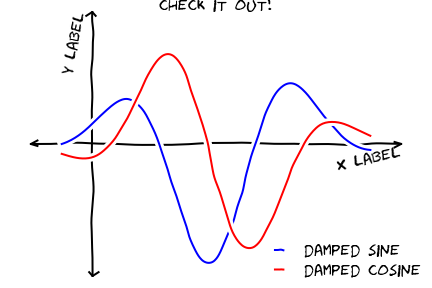Sometimes when showing schematic plots, this is the type of figure I want to display. But drawing it by hand is a pain: I'd rather just use matplotlib. The problem is, matplotlib is a bit too precise. Attempting to duplicate this figure in matplotlib leads to something like this:

In :
Image('http://jakevdp.github.com/figures/mpl_version.png')

Out: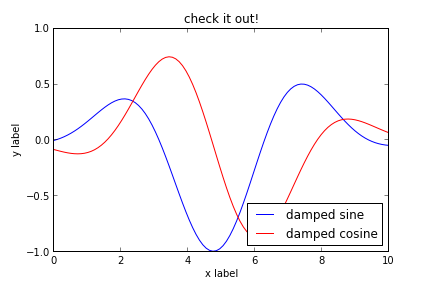It just doesn't have the same effect. Matplotlib is great for scientific plots, but sometimes you don't want to be so precise.

This subject has recently come up on the matplotlib mailing list, and started some interesting discussions. As near as I can tell, this started with a thread on a mathematica list which prompted a thread on the matplotlib list wondering if the same could be done in matplotlib.

Damon McDougall offered a quick solution which was improved by Fernando Perez in this notebook, and within a few days there was a matplotlib pull request offering a very general way to create sketch-style plots in matplotlib. Only a few days from a cool idea to a working implementation: this is one of the most incredible aspects of package development on github.

The pull request looks really nice, but will likely not be included in a released version of matplotlib until at least version 1.3. In the mean-time, I wanted a way to play around with these types of plots in a way that is compatible with the current release of matplotlib. To do that, I created the following code:

## The Code: XKCDify¶

XKCDify will take a matplotlib Axes instance, and modify the plot elements in-place to make them look hand-drawn. First off, we'll need to make sure we have the Humor Sans font. It can be downloaded using the command below.

Next we'll create a function xkcd_line to add jitter to lines. We want this to be very general, so we'll normalize the size of the lines, and use a low-pass filter to add correlated noise, perpendicular to the direction of the line. There are a few parameters for this filter that can be tweaked to customize the appearance of the jitter.

Finally, we'll create a function which accepts a matplotlib axis, and calls xkcd_line on all lines in the axis. Additionally, we'll switch the font of all text in the axes, and add some background lines for a nice effect where lines cross. We'll also draw axes, and move the axes labels and titles to the appropriate location.

In :
"""
XKCD plot generator
-------------------
Author: Jake Vanderplas

This is a script that will take any matplotlib line diagram, and convert it
to an XKCD-style plot.  It will work for plots with line & text elements,
including axes labels and titles (but not axes tick labels).

The idea for this comes from work by Damon McDougall
http://www.mail-archive.com/[email protected]/msg25499.html
"""
import numpy as np
import pylab as pl
from scipy import interpolate, signal
import matplotlib.font_manager as fm

# We need a special font for the code below.  It can be downloaded this way:
import os
import urllib2
if not os.path.exists('Humor-Sans.ttf'):

def xkcd_line(x, y, xlim=None, ylim=None,
mag=1.0, f1=30, f2=0.05, f3=15):
"""
Mimic a hand-drawn line from (x, y) data

Parameters
----------
x, y : array_like
arrays to be modified
xlim, ylim : data range
the assumed plot range for the modification.  If not specified,
they will be guessed from the  data
mag : float
magnitude of distortions
f1, f2, f3 : int, float, int
filtering parameters.  f1 gives the size of the window, f2 gives
the high-frequency cutoff, f3 gives the size of the filter

Returns
-------
x, y : ndarrays
The modified lines
"""
x = np.asarray(x)
y = np.asarray(y)

# get limits for rescaling
if xlim is None:
xlim = (x.min(), x.max())
if ylim is None:
ylim = (y.min(), y.max())

if xlim == xlim:
xlim = ylim

if ylim == ylim:
ylim = xlim

# scale the data
x_scaled = (x - xlim) * 1. / (xlim - xlim)
y_scaled = (y - ylim) * 1. / (ylim - ylim)

# compute the total distance along the path
dx = x_scaled[1:] - x_scaled[:-1]
dy = y_scaled[1:] - y_scaled[:-1]
dist_tot = np.sum(np.sqrt(dx * dx + dy * dy))

# number of interpolated points is proportional to the distance
Nu = int(200 * dist_tot)
u = np.arange(-1, Nu + 1) * 1. / (Nu - 1)

# interpolate curve at sampled points
k = min(3, len(x) - 1)
res = interpolate.splprep([x_scaled, y_scaled], s=0, k=k)
x_int, y_int = interpolate.splev(u, res)

# we'll perturb perpendicular to the drawn line
dx = x_int[2:] - x_int[:-2]
dy = y_int[2:] - y_int[:-2]
dist = np.sqrt(dx * dx + dy * dy)

# create a filtered perturbation
coeffs = mag * np.random.normal(0, 0.01, len(x_int) - 2)
b = signal.firwin(f1, f2 * dist_tot, window=('kaiser', f3))
response = signal.lfilter(b, 1, coeffs)

x_int[1:-1] += response * dy / dist
y_int[1:-1] += response * dx / dist

# un-scale data
x_int = x_int[1:-1] * (xlim - xlim) + xlim
y_int = y_int[1:-1] * (ylim - ylim) + ylim

return x_int, y_int

def XKCDify(ax, mag=1.0,
f1=50, f2=0.01, f3=15,
bgcolor='w',
xaxis_loc=None,
yaxis_loc=None,
xaxis_arrow='+',
yaxis_arrow='+',
ax_extend=0.1,
expand_axes=False):
"""Make axis look hand-drawn

This adjusts all lines, text, legends, and axes in the figure to look
like xkcd plots.  Other plot elements are not modified.

Parameters
----------
ax : Axes instance
the axes to be modified.
mag : float
the magnitude of the distortion
f1, f2, f3 : int, float, int
filtering parameters.  f1 gives the size of the window, f2 gives
the high-frequency cutoff, f3 gives the size of the filter
xaxis_loc, yaxis_log : float
The locations to draw the x and y axes.  If not specified, they
will be drawn from the bottom left of the plot
xaxis_arrow, yaxis_arrow : str
where to draw arrows on the x/y axes.  Options are '+', '-', '+-', or ''
ax_extend : float
How far (fractionally) to extend the drawn axes beyond the original
axes limits
expand_axes : bool
if True, then expand axes to fill the figure (useful if there is only
a single axes in the figure)
"""
# Get axes aspect
ext = ax.get_window_extent().extents
aspect = (ext - ext) / (ext - ext)

xlim = ax.get_xlim()
ylim = ax.get_ylim()

xspan = xlim - xlim
yspan = ylim - xlim

xax_lim = (xlim - ax_extend * xspan,
xlim + ax_extend * xspan)
yax_lim = (ylim - ax_extend * yspan,
ylim + ax_extend * yspan)

if xaxis_loc is None:
xaxis_loc = ylim

if yaxis_loc is None:
yaxis_loc = xlim

# Draw axes
xaxis = pl.Line2D([xax_lim, xax_lim], [xaxis_loc, xaxis_loc],
linestyle='-', color='k')
yaxis = pl.Line2D([yaxis_loc, yaxis_loc], [yax_lim, yax_lim],
linestyle='-', color='k')

# Label axes3, 0.5, 'hello', fontsize=14)
ax.text(xax_lim, xaxis_loc - 0.02 * yspan, ax.get_xlabel(),
fontsize=14, ha='right', va='top', rotation=12)
ax.text(yaxis_loc - 0.02 * xspan, yax_lim, ax.get_ylabel(),
fontsize=14, ha='right', va='top', rotation=78)
ax.set_xlabel('')
ax.set_ylabel('')

ax.text(0.5 * (xax_lim + xax_lim), yax_lim,
ax.get_title(),
ha='center', va='bottom', fontsize=16)
ax.set_title('')

Nlines = len(ax.lines)
lines = [xaxis, yaxis] + [ax.lines.pop(0) for i in range(Nlines)]

for line in lines:
x, y = line.get_data()

x_int, y_int = xkcd_line(x, y, xlim, ylim,
mag, f1, f2, f3)

# create foreground and background line
lw = line.get_linewidth()
line.set_linewidth(2 * lw)
line.set_data(x_int, y_int)

# don't add background line for axes
if (line is not xaxis) and (line is not yaxis):
line_bg = pl.Line2D(x_int, y_int, color=bgcolor,
linewidth=8 * lw)

# Draw arrow-heads at the end of axes lines
arr1 = 0.03 * np.array([-1, 0, -1])
arr2 = 0.02 * np.array([-1, 0, 1])

arr1[::2] += np.random.normal(0, 0.005, 2)
arr2[::2] += np.random.normal(0, 0.005, 2)

x, y = xaxis.get_data()
if '+' in str(xaxis_arrow):
ax.plot(x[-1] + arr1 * xspan * aspect,
y[-1] + arr2 * yspan,
color='k', lw=2)
if '-' in str(xaxis_arrow):
ax.plot(x - arr1 * xspan * aspect,
y - arr2 * yspan,
color='k', lw=2)

x, y = yaxis.get_data()
if '+' in str(yaxis_arrow):
ax.plot(x[-1] + arr2 * xspan * aspect,
y[-1] + arr1 * yspan,
color='k', lw=2)
if '-' in str(yaxis_arrow):
ax.plot(x - arr2 * xspan * aspect,
y - arr1 * yspan,
color='k', lw=2)

# Change all the fonts to humor-sans.
prop = fm.FontProperties(fname='Humor-Sans.ttf', size=16)
for text in ax.texts:
text.set_fontproperties(prop)

# modify legend
leg = ax.get_legend()
if leg is not None:
leg.set_frame_on(False)

for child in leg.get_children():
if isinstance(child, pl.Line2D):
x, y = child.get_data()
child.set_data(xkcd_line(x, y, mag=10, f1=100, f2=0.001))
child.set_linewidth(2 * child.get_linewidth())
if isinstance(child, pl.Text):
child.set_fontproperties(prop)

# Set the axis limits
ax.set_xlim(xax_lim - 0.1 * xspan,
xax_lim + 0.1 * xspan)
ax.set_ylim(yax_lim - 0.1 * yspan,
yax_lim + 0.1 * yspan)

ax.set_xticks([])
ax.set_yticks([])

if expand_axes:
ax.figure.set_facecolor(bgcolor)
ax.set_axis_off()
ax.set_position([0, 0, 1, 1])

return ax


## Testing it Out¶

Let's test this out with a simple plot. We'll plot two curves, add some labels, and then call XKCDify on the axis. I think the results are pretty nice!

In :
%pylab inline

Welcome to pylab, a matplotlib-based Python environment [backend: module://IPython.zmq.pylab.backend_inline].

In :
np.random.seed(0)

ax = pylab.axes()

x = np.linspace(0, 10, 100)
ax.plot(x, np.sin(x) * np.exp(-0.1 * (x - 5) ** 2), 'b', lw=1, label='damped sine')
ax.plot(x, -np.cos(x) * np.exp(-0.1 * (x - 5) ** 2), 'r', lw=1, label='damped cosine')

ax.set_title('check it out!')
ax.set_xlabel('x label')
ax.set_ylabel('y label')

ax.legend(loc='lower right')

ax.set_xlim(0, 10)
ax.set_ylim(-1.0, 1.0)

#XKCDify the axes -- this operates in-place
XKCDify(ax, xaxis_loc=0.0, yaxis_loc=1.0,
xaxis_arrow='+-', yaxis_arrow='+-',
expand_axes=True)

Out:
<matplotlib.axes.AxesSubplot at 0x2fecbd0>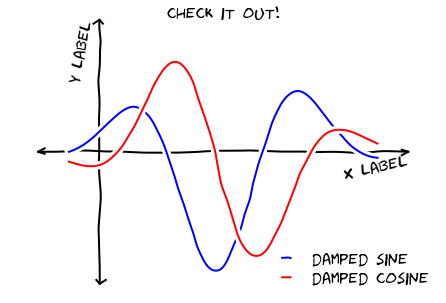## Duplicating an XKCD Comic¶

Now let's see if we can use this to replicated an XKCD comic in matplotlib. This is a good one:

In :
Image('http://imgs.xkcd.com/comics/front_door.png')

Out: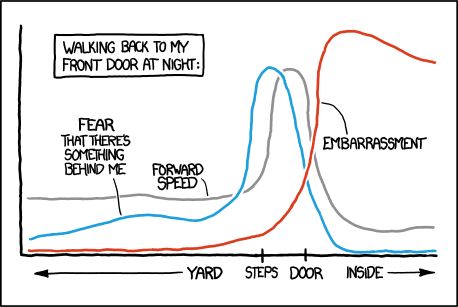With the new XKCDify function, this is relatively easy to replicate. The results are not exactly identical, but I think it definitely gets the point across!

In :
# Some helper functions
def norm(x, x0, sigma):
return np.exp(-0.5 * (x - x0) ** 2 / sigma ** 2)

def sigmoid(x, x0, alpha):
return 1. / (1. + np.exp(- (x - x0) / alpha))

# define the curves
x = np.linspace(0, 1, 100)
y1 = np.sqrt(norm(x, 0.7, 0.05)) + 0.2 * (1.5 - sigmoid(x, 0.8, 0.05))

y2 = 0.2 * norm(x, 0.5, 0.2) + np.sqrt(norm(x, 0.6, 0.05)) + 0.1 * (1 - sigmoid(x, 0.75, 0.05))

y3 = 0.05 + 1.4 * norm(x, 0.85, 0.08)
y3[x > 0.85] = 0.05 + 1.4 * norm(x[x > 0.85], 0.85, 0.3)

# draw the curves
ax = pl.axes()
ax.plot(x, y1, c='gray')
ax.plot(x, y2, c='blue')
ax.plot(x, y3, c='red')

ax.text(0.3, -0.1, "Yard")
ax.text(0.5, -0.1, "Steps")
ax.text(0.7, -0.1, "Door")
ax.text(0.9, -0.1, "Inside")

ax.text(0.05, 1.1, "fear that\nthere's\nsomething\nbehind me")
ax.plot([0.15, 0.2], [1.0, 0.2], '-k', lw=0.5)

ax.text(0.25, 0.8, "forward\nspeed")
ax.plot([0.32, 0.35], [0.75, 0.35], '-k', lw=0.5)

ax.text(0.9, 0.4, "embarrassment")
ax.plot([1.0, 0.8], [0.55, 1.05], '-k', lw=0.5)

ax.set_title("Walking back to my\nfront door at night:")

ax.set_xlim(0, 1)
ax.set_ylim(0, 1.5)

# modify all the axes elements in-place
XKCDify(ax, expand_axes=True)

Out:
<matplotlib.axes.AxesSubplot at 0x2fef210>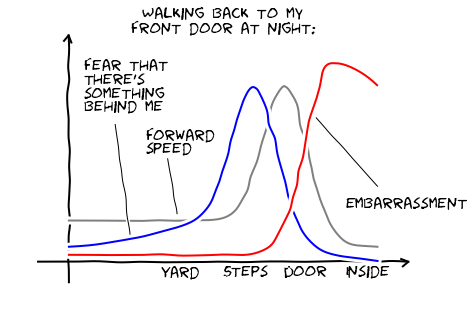Pretty good for a couple hours's work!

I think the possibilities here are pretty limitless: this is going to be a hugely useful and popular feature in matplotlib, especially when the sketch artist PR is mature and part of the main package. I imagine using this style of plot for schematic figures in presentations where the normal crisp matplotlib lines look a bit too "scientific". I'm giving a few talks at the end of the month... maybe I'll even use some of this code there.

This post was written entirely in an IPython Notebook: the notebook file is available for download here. For more information on blogging with notebooks in octopress, see my previous post on the subject.